# Some New Classes of Three Dimensional Fractals

Wed 17 August 2016

As discussed in printing fractals. (which you may want to read if you haven't done so), in the mandelbulb the concept of complex exponentiation is extended into three dimensions. If a number is raised to the power N an {x,y,z} triplet is converted into spherical coordinates, the magnitude of the vector (r) is raised to N, and the spherical angles $$\theta$$ and $$\phi$$ are multiplied by N: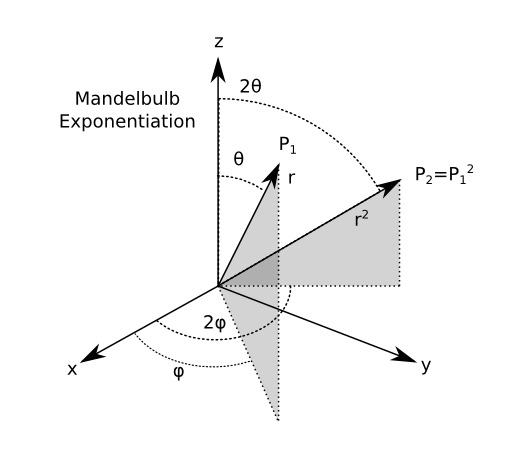But there are other ways that the concept can be extended.

For the work presented here, multicomplex (or, specifically, tricomplex) numbers are employed. Briefly: In normal complex arithmetic a number is defined by the pair {x,y} as

\begin{equation*} z = (x+iy) \end{equation*}

where i is $$\sqrt{-1}$$:

\begin{equation*} i \cdot i = -1 \end{equation*}

This concept can be extended to three dimensions such that

\begin{equation*} z = (x+iy+jz) \end{equation*}

where

\begin{align*} i \cdot i &= j \\ j \cdot j &= i \\ i \cdot j &= 1 \end{align*}

(Note that multiplication is communitive, so ij = ji.) With these definitions we can see that additions and subtraction are pair-wise, and multiplication is given by the following identity:

\begin{equation*} z \cdot z' = \{(xx')+(yz')+(zy'),(xy')+(yx')+(zz'),(xz')+ (zx') + (yy')\} \end{equation*}

where

\begin{align*} z &= {x+iy+jz} \\ z' &= {x'+iy'+jz'} \end{align*}

## Reference Vector Exponentiation

One such extension involves rotation about a reference vector (or vectors):

1. A reference vector is defined
2. An axis of rotation is calculated by taking the cross product of the reference vector and the point being exponentiated
3. The magnitude of the point vector is raised by N
4. The angle $$\theta$$ between the reference vector and the point vector is multiplied by N.

This is shown graphically (for N=2) below: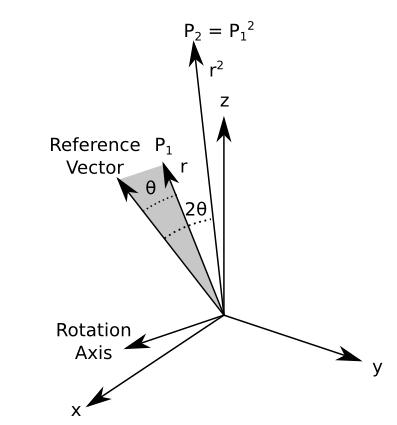With one reference vector defined the structures produced have radial symmetry but do not exhibit anything too aesthetically extraordinary.

As an example, we can define our structure:

\begin{align*} z_{n+1} &= z_{n}^4 + c \\ V_{\text{ref}} &= [1,0,0] \end{align*}

which produces the following output: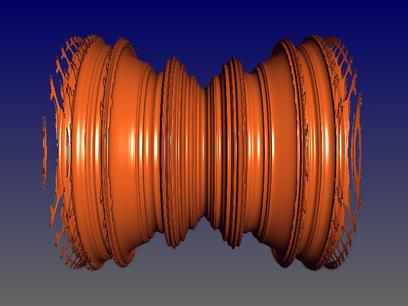(Click to Enlarge)

(It should be noted that the disconnected regions at the end could be rendering artifacts. It's possible that the figure is connected.)

However, if we define two reference vectors (producing two exponentiations/rotations) the figure exhibits much more structure. Given the definition

\begin{align*} z_{n+1} &= z_{n}^4 + c \\ V_{\text{ref}} &= [1,0,0],[0,1,0] \end{align*}

the structure produced is: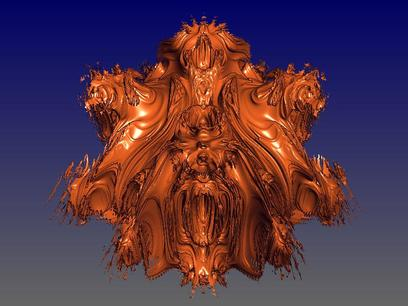(Click to Enlarge)

When three axes are added, i.e

\begin{equation*} V_{\text{ref}} = [1,0,0],[0,1,0],[0,0,1] \end{equation*}

we end up with even more structure: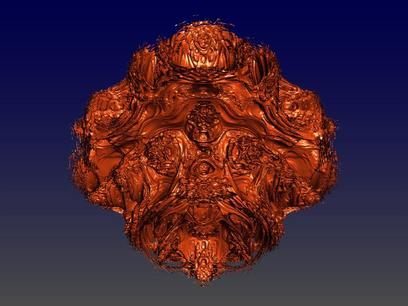(Click to Enlarge)

### Julia Representations

Recall that with the standard Mandelbrot set

\begin{equation*} z_{n+1} = z_{n}^2 + c \end{equation*}

the initial z is given as {0 + 0i} and c is taken from the coordinate in question, but that it is also possible to assign a constant to c and initialize z to the point in question ({x + yi}), yielding the julia set. The same thing, of course, can also be done with the equations proposed here.

For brevity I will show c as a real constant below. For the initial explorations the I used the following definition to transform the real constant to a tricomplex number:

\begin{equation*} c = \{a,ai,aj\} \end{equation*}

where a is the real constant.

The julia representation of the two-axis figure shows much more structure than the Mandelbrot-ish render:

\begin{align*} z_{n+1} &= z_{n}^4 + c \\ c &= 0.4 \\ V_{\text{ref}} &= [1,0,0],[0,1,0] \end{align*}[Click to Enlarge]

The three-axis figure shows more structure still:

\begin{align*} z_{n+1} &= z_{n}^4 + c \\ c &= 0.375 \\ V_{\text{ref}} &= [1,0,0],[0,1,0],[0,0,1] \end{align*}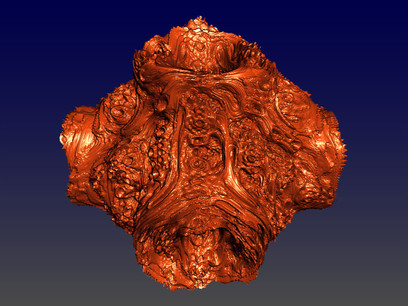[Click to Enlarge]

### Self-Similarity

These structures show much detail considering the brevity with which they are defined, but are they fractal in structure? To investigate this we can ask a related question: do they exhibit self-similar detail at all scales?

The following slide-show shows successive zoom into the two-axis structure, with the approximate area of zoom for each figure shown in the preceding one.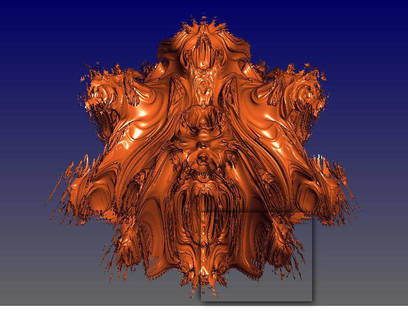Click For Zoom

It's hard to say for sure (especially considering the rendering artifacts -- rendering these structures is non-trivial numerically), but it appears as if this figure may bottom out. In other words, detail may decrease as scale decreases.

However, looks can be deceiving, and when rendering such structures there are always practical considerations: formally, a point is in the set if it always stays bounded, but to determine this a certain number of iterations will be preformed. Higher levels of iteration more finely define the structure surface, but they take longer to calculate and they can produce rougher surfaces that are more difficult to render cleanly. Thus one always must make trade offs between tractability, clarity, and accuracy.

In the figures shown above the maximum iteration count was 5. If we take the image of 2-axis structure and increase the maximum iteration count to 10 we obtain the following picture: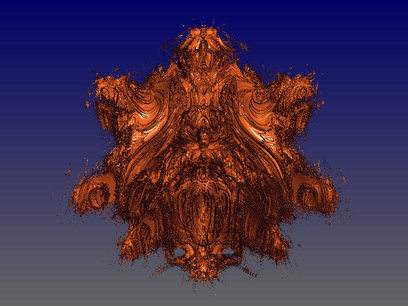Two Axis, Maximum Interations = 10

It's still impossible to say, but the situation is a lot more ambiguous now. There is a good deal more detail when the extra iterations are performed, and infinite detail is not beyond the realm of possibility. But there also appears to be many more rendering artifacts, and it is difficult to get a clean render of this figure at high iteration.

So we'll examine some of the structures which appear to offer more detail from the outset, starting this the julia-style render of the two-axis figure. Based upon the initial renders the julia-style calculations appear to be a bit more stable and well-behaved, so there should be fewer artifacts that may hide lower-level details.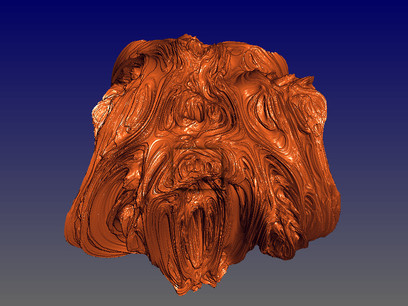Two Axis, Julia Rendering

As seen above, there doesn't seem to be a lessening of detail at smaller scale. This is perhaps even more striking with the three-axis julia render:Three-Axis Julia Zoom

There is no sign of a lessening of detail; all appearances are that self-similarity continues to be exhibited at all scales and levels. In the sequence of images above the highest zoom level has a field of view about 4100 times smaller than the initial image, with an area about 17 million times smaller. Even at this level of magnification no lessening of detail can be observed. While this is not a mathematical proof, it seems reasonable to assert that this figure exhibits a fractal nature.